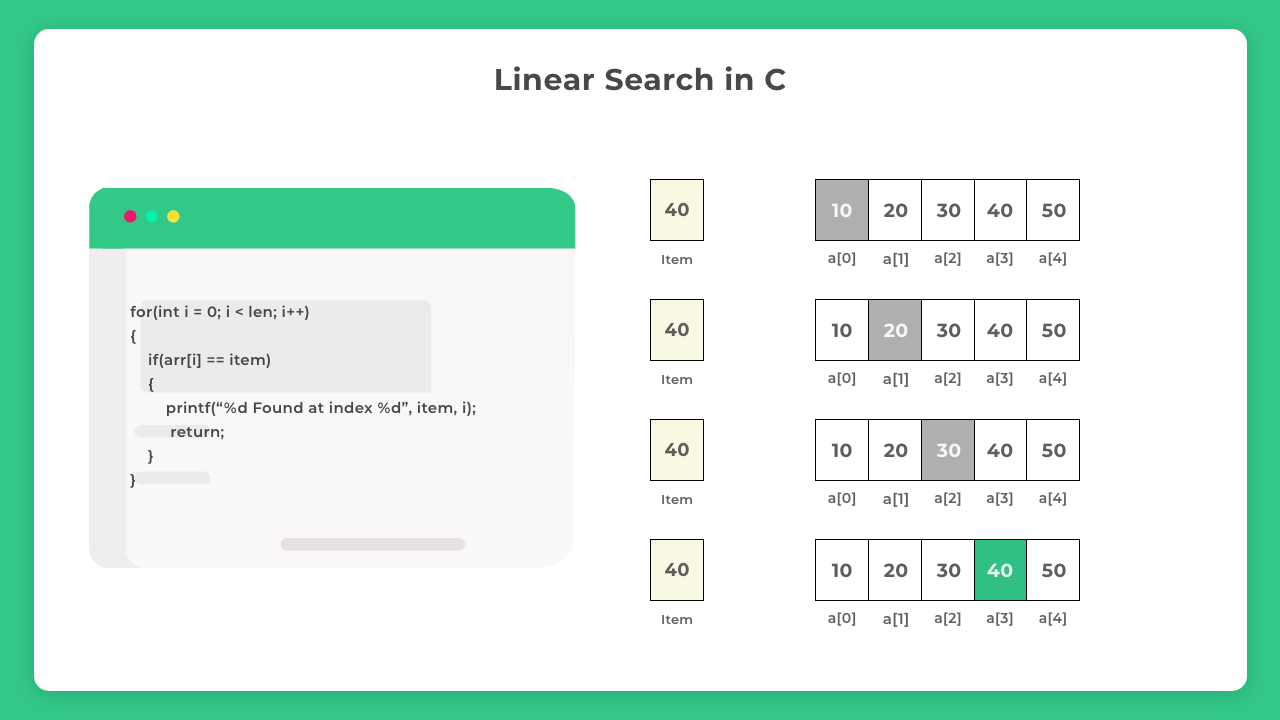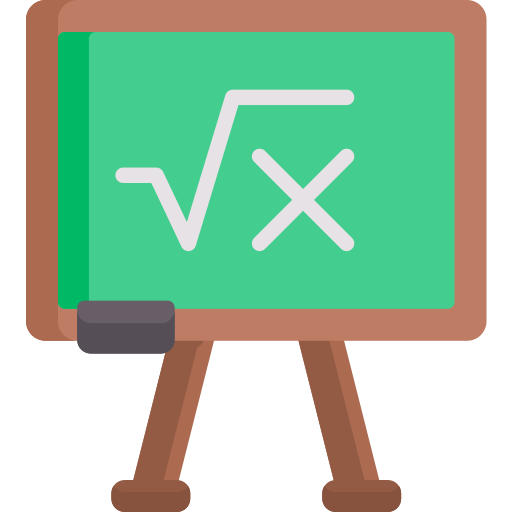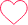# Linear Search in C

## Linear Search in C

Searching, in normal ways, can be coined as” to find the hidden thing”. In data structure.the searching algorithm is used to find whether a given number is present and if it is present then at what location it occurs.

There are two types of searching algorithm present in data structure through which searching any data become more easy.

1. Linear search or sequential search
2. Binary searchTime Complexity O(n) Best Case O(1) Worst Case O(n) Space Complexity O(1) Avg. Comparisons (n+1)/2

## Implementation:-

• Step 1- Take array input and calculate the number of elements in the array call this as arr[]
• Step 2- Take the input for the item to be searched in the array. Call this as item
• Step 3- Linearly traverse the array using a for loop.
• Step 4 –  For each array item check if arr[i] == item
• If such condition is met print value and its position and return to the main function
• If no such element is found and the whole array is traversed. Print not found## C Code:-

Run
```#include <stdio.h>

void LinearSearch(int arr[], int len, int item)
{
for(int i=0;i < len;i++)
{
if(arr[i] == item)
{
printf("%d Found at index %d", item, i);
return;
}
}
}

int main()
{
int arr[] = {10, 20, 30, 40, 50};

// calculating length of array
int len = sizeof(arr)/sizeof(arr);

// item to be searched
int item = 40;
LinearSearch(arr, len, item);

return 0;
}```

### Output:-

`40 Found at index 3`

### Pros

1. Very easy to understand and implement
2. Quick to write the code
3. Ideal for Unsorted array
4. Ideal for Array with lesser number of items

### Cons

1. Time complexity is bad O(n)
2. Binary Search gives better, efficient and faster results## Using Recursion

This method uses recursion in C.

Run
```// Time Complexity : O(N)
// Space Complexity : O(1)
// Auxiliary Space Complexity : O(N) due to function call stack
#include<stdio.h>

int LinearSearch(int arr[], int index, int item)
{
if(arr[index] == item)
return index;
else if (index == -1)
return -1;

LinearSearch(arr, index - 1, item);
}

int main()
{
int arr[] = {10, 20, 30, 40, 50};

// calculating length of array
int len = sizeof(arr)/sizeof(arr);

// item to be searched
int item = 30;

int index = LinearSearch(arr, len - 1, item);

if(index >= 0)
printf("Item found at %d index", index);
else

return 0;
}```

### Output

`Item found at 2 index`

O(1)

O(n)

O(n)

O(1)

(n+1)/2

O(1)

O(n)

O(n)

O(1)

## Average Comparisons

(n+1)/2### Quiz TimeQuestion 1. The average number of key comparisons done in a successful sequential search in a list of length is –

1. (n-1)/2
2. Log n
3. (n+1)/2
4. (n)/2

(Amazon – Mettl Test)

Just say if you have to find a given element in a sequential search. It can be found in the first try, then 1 comparison is required similarly…total comparisons can be 1+2+…+n = n(n+1)/2

Avg will be n(n+1)/2 divided by n ( total elements) = (n+1)/2

Ans. Option CQuestion 1. The average number of key comparisons done in a successful sequential search in a list of length is –

1. (n-1)/2
2. Log n
3. (n+1)/2
4. (n)/2

(Amazon – Mettl Test)

Just say if you have to find a given element in a sequential search. It can be found in the first try, then 1 comparison is required similarly…total comparisons can be 1+2+…+n = n(n+1)/2

Avg will be n(n+1)/2 divided by n ( total elements) = (n+1)/2

Ans. Option CQuestion 1. What is the best case for linear search?

1. O(nlogn)
2. O(long)
3. O(n)
4. O(1)

(Wipro – AMCAT Test)

If the item is present at the first index only then the time complexity in this best case is O(1).Question 3. The worst-case occurred in the linear search algorithm when
1. The element in the middle of an array
2. Item present in the last
3. Item present in the starting
4. Item has the maximum value

(TCS NQT)

If the element situated at the end of the array, so it takes maximum time to search for that of the element.

Ans. Option BQuestion 4. What is the recurrence relation for the linear search recursive algorithm?

1. T(n-2)+c
2. 2T(n-1)+c
3. T(n-1)+c
4. T(n+1)+c

(TCS NQT)

Since after each recursive call the size is reduced by 1 each time thus.

Ans. Option C

### Asked in the following exams/interviews## Searching

Searching algorithms are easy to learn but are really important for college semester exams and companies offering package between 3 – 6 LPA would ask direct searching questions in online test/ interviews.

### 7 comments on “Linear Search in C”

•6008

thank you so much ,it’s really very helpful1
•Amar

awesome1
•himanshu

nice2
•Jaspreet2
•Care

Thanks a ton, for the appreciation jaspreet, you must visit our Linked List section in the Data Structure Page, you will find some good content there too – https://prepinsta.com/data-structures/#linked2
•himanshu

nice2
•Anugya Saxena

thanks for these study material in summary with pictorial representation.5